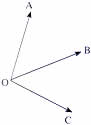Thursday , July 7 2022# NCERT 5th Class (CBSE) Mathematics: Lines And Angle

## ANGLES

### Types of Angles

Right, acute, obtuse and straight angles are all types of angles that you have studied so far. There are two more types of angles.

Reflex angle: Look at the railing of this staircase. They from an angle on the outside that is more than 180°.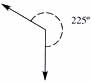#### An angle that is more than 180° and less than 360° is called a reflex angle.

Whole angle: If we draw several angles around a point and measure and add them, we find that they always add up to 360°.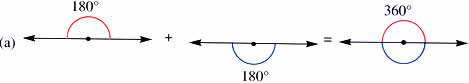(b) 100 + 45 + 45 + 50 + 120 = 360°

A whole angle measures 360°.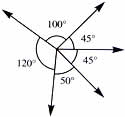### Measuring Reflex Angles

we can use our knowledge of whole angles to measure reflex angles.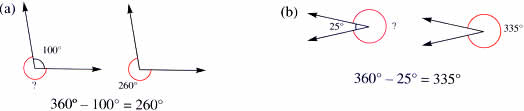### Angle Relationships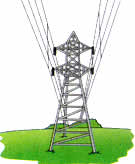This electric power line reminds us of many angles and the relationship between them.